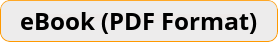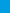# SAT Math Prep 2019 A Comprehensive Review and Ultimate Guide to the SAT Math TestSAT Math Prep 2019 provides students with the confidence and math skills they need to succeed on the SAT Math, building a solid foundation of basic Math topics with abundant exercises for each topic. It is designed to address the needs of SAT test takers who must have a working knowledge of basic Math.\$12.99
-28% only \$12.99
Rated 4.86 out of 5 based on 7 customer ratings

Bulk Orders: Need a license for this book?

The Only Book You’ll Ever Need to ACE the SAT Math Test!

SAT Math Prep 2019 provides students with the confidence and math skills they need to succeed on the SAT Math, building a solid foundation of basic Math topics with abundant exercises for each topic. It is designed to address the needs of SAT test takers who must have a working knowledge of basic Math.

This comprehensive book with over 2,500 sample questions and 2 complete SAT tests is all you need to fully prepare for the SAT Math. It will help you learn everything you need to ace the math section of the SAT.

There are more than 2,500 Math problems with answers in this book.

Effortless Math unique study program provides you with an in-depth focus on the math portion of the exam, helping you master the math skills that students find the most troublesome.

This book contains most common sample questions that are most likely to appear in the math section of the SAT.

Inside the pages of this comprehensive SAT Math book, students can learn basic math operations in a structured manner with a complete study program to help them understand essential math skills. It also has many exciting features, including:

• Dynamic design and easy-to-follow activities
• A fun, interactive and concrete learning process
• Targeted, skill-building practices
• Fun exercises that build confidence
• Math topics are grouped by category, so you can focus on the topics you struggle on
• All solutions for the exercises are included, so you will always find the answers
• 2 Complete SAT Math Practice Tests that reflect the format and question types on SAT

SAT Math Prep 2019 is an incredibly useful tool for those who want to review all topics being covered on the SAT test. It efficiently and effectively reinforces learning outcomes through engaging questions and repeated practice, helping you to quickly master basic Math skills.

Get a copy today and see how fast you will prepare for the test with the SAT Mathematics Prep 2019!

Effortless Math Education
www.effortlessmath.com

## 7 reviews for SAT Math Prep 2019

1.Rated 5 out of 5

Great book!

2.Rated 5 out of 5

Best Math SAT workbook on the market, hands down.

3.Rated 5 out of 5

I love how the concepts are broken down in the this book, and it has plenty of exercises and practice problems.

4.Rated 5 out of 5

This is the most beneficial of the several SAT math books we tried. The explanations are clear, and the questions seem relevant to the real SAT Math test.

5.Rated 5 out of 5

Good Math questions for my son.

6.Rated 4 out of 5

Good book!

7.Rated 5 out of 5

I 100% recommend this book for those preparing for the SAT Math test. Although this book is for those who have already learned the basic concepts of arithmetic, algebra, statistics and geometry, it is useful.

?